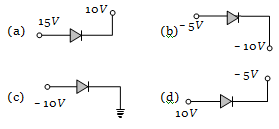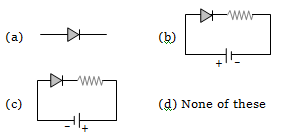In a PN-junction diode not connected to any circuit

(a) The potential is the same everywhere

(b) The P-type is at higher potential than the N-type side

(c) There is an electric field at the junction directed from the N- type side to the P- type side

(d) There is an electric field at the junction directed from the P-type side to the N-type side

Concept Questions :-

PN junction
High Yielding Test Series + Question Bank - NEET 2020

Difficulty Level:

Which of the following statements is not true

(a) The resistance of intrinsic semiconductors decrease with increase of temperature

(b) Doping pure with trivalent impurities give P-type semiconductors

(c) The majority carriers in N-type semiconductors are holes

(d) A PN-junction can act as a semiconductor diode

Concept Questions :-

Types of semiconductors
High Yielding Test Series + Question Bank - NEET 2020

Difficulty Level:

The dominant mechanisms for motion of charge carriers in forward and reverse biased silicon P-N junctions are

(a) Drift in forward bias, diffusion in reverse bias

(b) Diffusion in forward bias, drift in reverse bias

(c) Diffusion in both forward and reverse bias

(d) Drift in both forward and reverse bias

Concept Questions :-

PN junction
High Yielding Test Series + Question Bank - NEET 2020

Difficulty Level:

Which one is reverse-biasedConcept Questions :-

Applications of PN junction
High Yielding Test Series + Question Bank - NEET 2020

Difficulty Level:

Which one is in forward biasConcept Questions :-

PN junction
High Yielding Test Series + Question Bank - NEET 2020

Difficulty Level:

The reason of current flow in P-N junction in forward bias is

(a) Drifting of charge carriers

(b) Minority charge carriers

(c) Diffusion of charge carriers

(d) All of these

Concept Questions :-

PN junction
High Yielding Test Series + Question Bank - NEET 2020

Difficulty Level:

The resistance of a reverse biased P-N junction diode is about
(a) 1 ohm                        (b) ${10}^{2}$ohm
(c) ${10}^{3}$ ohm                     (d) ${10}^{6}$ ohm

Concept Questions :-

PN junction
High Yielding Test Series + Question Bank - NEET 2020

Difficulty Level:

Avalanche breakdown is due to

(a) Collision of minority charge carrier

(b) Increase in depletion layer thickness

(c) Decrease in depletion layer thickness

(d) None of these

Concept Questions :-

Energy band theory
High Yielding Test Series + Question Bank - NEET 2020

Difficulty Level:

Zener breakdown in a semi-conductor diode occurs when

(a) Forward current exceeds certain value

(b) Reverse bias exceeds certain value

(c) Forward bias exceeds certain value

(d) Potential barrier is reduced to zero

Concept Questions :-

Applications of PN junction
High Yielding Test Series + Question Bank - NEET 2020

Difficulty Level:

When forward bias is applied to a P-N junction, then what happens to the potential barrier ${V}_{\mathbit{B}}$, and the width of charge depleted region x

(a) ${V}_{\mathbit{B}}$ increases, x decreases

(b) ${V}_{\mathbit{B}}$ decreases, x increases

(c) ${V}_{\mathbit{B}}$ increases, x increases

(d) ${V}_{\mathbit{B}}$ decreases, x decreases

Concept Questions :-

PN junction